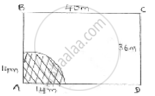Share

# A Horse is Placed for Grazing Inside a Rectangular Field 40m by 36m and is Tethered to One Corner by a Rope 14m Long. Over How Much Area Can It Graze. - CBSE Class 10 - Mathematics

ConceptPerimeter and Area of a Circle

#### Question

A horse is placed for grazing inside a rectangular field 40m by 36m and is tethered to one corner by a rope 14m long. Over how much area can it graze.

#### SolutionThe fig shows rectangular field ABCD at corner A, a horse is tied with rope length = 14m.

The area it can graze is represented A as shaded region= area of quadrant with (radius = length) of string

Area = 1/4× (𝑎𝑟𝑒𝑎 𝑜𝑓 𝑐𝑖𝑟𝑐𝑙𝑒) = 𝜋𝑟2

= 1/4 ×22/7 × 14 × 14

= (22 × 7)

= 154 m2.

Area it can graze = 154m2.

Is there an error in this question or solution?

#### Video TutorialsVIEW ALL 

Solution A Horse is Placed for Grazing Inside a Rectangular Field 40m by 36m and is Tethered to One Corner by a Rope 14m Long. Over How Much Area Can It Graze. Concept: Perimeter and Area of a Circle.
S